Equations
Exponents
Order of Operations
Fractions & Decimals
Percent
Misc.
100

5x=-105

-21

100

2^4

16

100

Simplify the following expression:

66div3*2+5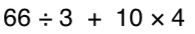49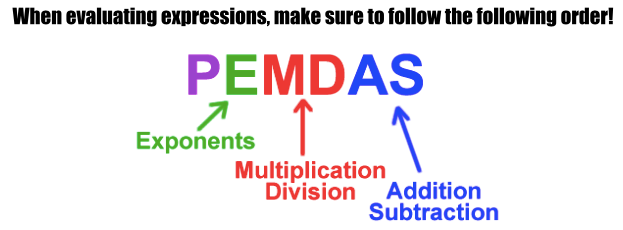100

-4 + -5

9100

Convert the following decimal to a fraction .38

17/50

100

What is 3/4 as a percent?

75%

100

What is the Prime factorization of 24

2*2*2*3

200

p-54=36

90

200

What is

9^3

729

200

Simplify the following expression:

9div3*3-5+7

11

200

14 + -12

2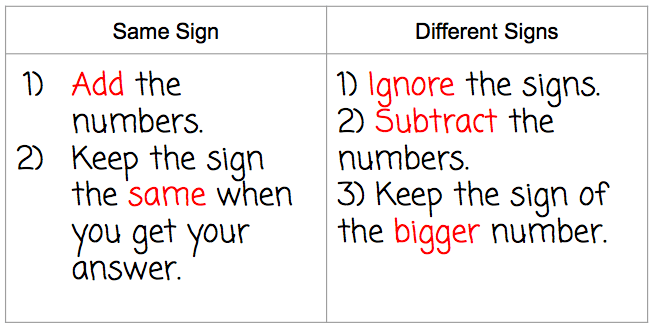200

What is 8/10 as a decimal?

0.8

200

50% of 297 is what number?

150

200

What 2 shapes do you see?

What 2 formulas will you use to find the TOTAL Area?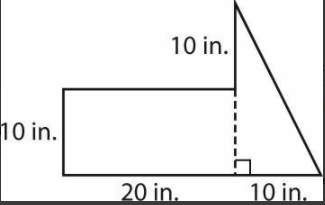Triangle and Rectangle

Area = 1/2bh

Area = bh or lw

300

1/2x=10

x=20

300

what is

2^5

32

300

3 + 42 * (3 + 2)

83300

-10 + 15

5300

Convert the following fraction to a decimal 7/20

.35

300

95% is what as a fraction in simplest form?

19/20

300

What is the greatest common factor of 64, 16, 32

16

400

k - 2.18=8

87.14

400

What is the value of:

84

4,096

400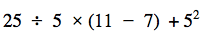45400

-18 + (-12) =

-30

400

3/4 + 1/6

11/12

400

What percent of 56 is 224?

400%

400

Find the Least common Multiple of 14, 21 and 7

42

500

8=k/2.5

x=20

50030500

6 + (-2 + -5)

-1

500

Divide the following

2/5 divided by 7/10

4/7

500

12.5 is what percent of 1250?

1%

500

Find the Least Common Multiple of 3, 12, 6

12

Click to zoom